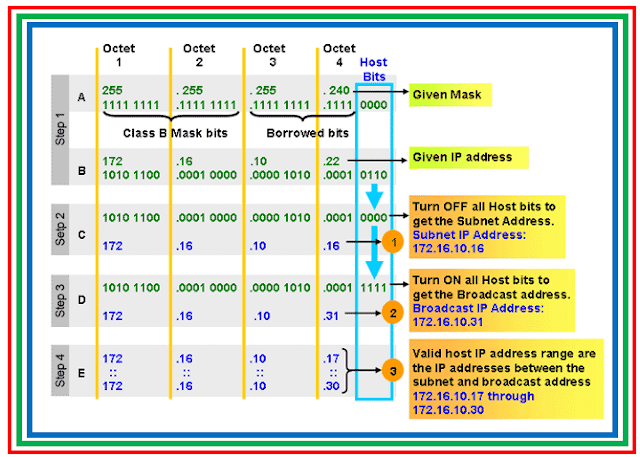Classless Inter-Domain Routing (CIDR) is a method for allocating IP addresses and routing Internet Protocol packets. The Internet Engineering Task Force introduced CIDR in 1993 to replace the previous addressing architecture of classful network design in the Internet. Its goal was to slow the growth of routing tables on routers across the Internet, and to help slow the rapid exhaustion of IPv4 addresses.

CIDR notation is a compact representation of an IP address and its associated routing prefix. The notation is constructed from the IP address and the prefix size, the latter being equivalent to the number of leading 1 bits in the routing prefix mask. The IP address is expressed according to the standards of IPv4 or IPv6.

It is followed by a separator character, the slash ('/') character, and the prefix size expressed as a decimal number.

Variable Length Subnet Masking (VLSM) is a way of further Subnetting a subnet. Using Variable Length Subnet Masking (VLSM) we can allocate IPv4 addresses to the subnets by the exact need.Fig 1.1- IP Addressing- VLSM

Variable Length Subnet Masking (VLSM) allows us to use more than one subnet mask within the same network address space. If we recollect from the previous lessons, we can divide a network only into subnets with equal number of IPv4 addresses.

Variable Length Subnet Masking (VLSM) allows to create subnets from a single network with unequal number of IPv4 addresses.

Example: We want to divide 192.168.10.0, which is a Class C network, into four networks, each with unequal number of IPv4 addresses requirements as shown below.

Subnet A : 126 IPv4 Addresses.
Subnet B : 62 IPv4 Addresses.
Subnet C : 30 IPv4 Addresses.
Subnet D : 30 IPv4 Addresses.

This type of division is not possible as described in previous lessons, since it divide the network equally, but is possible with Variable Length Subnet Masking (VLSM).

Original Network (Network to be subnetted) – 192.168.10.0/24

Variable Length Subnet Masking (VLSM) - First Division

Divide the two networks equally with 128 IPv4 addresses (126 usable IPv4 addresses) in each network using 255.255.255.128 subnet mask (192.168.10.0/25).

We will get two subnets each with 128 IPv4 addresses (126 usable IPv4 addresses).

1) 192.168.10.0/25, which can be represented in binaries as below.
11000000.10101000.00001010.00000000
11111111.11111111.11111111.10000000

2) 192.168.10.128/25, which can be represented in binaries as below.
11000000.10101000.00001010.10000000
11111111.11111111.11111111.10000000

Variable Length Subnet Masking (VLSM)- Second Division

Divide second subnet (192.168.10.128/25) we got from the first division again into two Networks, each with 64 IP Addresses (62 usable IPv4 addresses) using 255.255.255.192 subnet mask.

We will get two subnets each with 64 IPv4 addresses (62 usable IPv4 addresses).

1) 192.168.10.128/26, which can be represented in binaries as below.
11000000.10101000.00001010.10000000
11111111.11111111.11111111.11000000

2) 192.168.10.192/26
11000000.10101000.00001010.11 000000
11111111.11111111.11111111.11000000

Variable Length Subnet Masking (VLSM) - Third Division

Divide 192.168.10.192/26 Network again into two Networks, each with 32 IPv4 addresses (30 usable IPv4 addresses) using 255.255.255.224 subnet mask

We will get two subnets each with 32 IPv4 addresses (30 usable IPv4 addresses).

1) 192.168.10.192/27, which can be represented in binaries as below.
11000000.10101000.00001010.11000000
11111111.11111111.11111111.11100000

2) 192.168.10.224/27, which can be represented in binaries as below.
11000000.10101000.00001010.11100000
11111111.11111111.11111111.11100000

Now we have split the 192.168.10.0/24 network into four subnets using Variable Length Subnet Masking (VLSM), with unequal number of IPv4 addresses as shown below. Also note that when you divide a network using Variable Length Subnet Masking (VLSM), the subnet masks are also different.

1) 192.168.10.0 - 255.255.255.128 (126 (128-2) usable IPv4 addresses)
2) 192.168.10.128 - 255.255.255.192 (62 (64-2) usable IPv4 addresses)
3) 192.168.10.192 - 255.255.255.224 (30 (32-2) usable IPv4 addresses)
4) 192.168.10.224 - 255.255.255.224 (30 (32-2) usable IPv4 addresses)